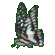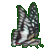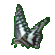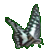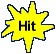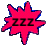Calculus Derivative Rules for Powers & Constants     Math Help Fun     Game Tips:

- A derivative in Calculus can be described as an instantaneous rate of change.
The slope of a curve at a point P is an example of a derivative.
The speed of a moving particle at a time T is an example of a derivative.

- A sample curve or motion can be represented by a relation such as y=x2   or the equivalent   f(x)=x2.
The derivative corresponding to y=x2   can be represented as  dy/dx = 2x    or   y' = 2x   or   f '(x) = 2x.
The three derivative symbols  dy/dx,  y' and  f '(x)  are in common use by various authors.

- Similarly, the relation represented as s=t3   or   s(t)=t3
has it's corresponding derivative represented as  ds/dt = 3t2    or   s'(t) = 3t2.

- A derivative power rule is used to get  dy/dx = 2x from the given relation y=x2.
The same derivative power rule is used to get  ds/dt = 3t2 from the given relation s(t)=t3.

- The following 3 derivative rules are used in this game, with n representing a positive integer.

1) In general, the derivative power rule for y=xn yields the derivative  dy/dx = nxn-1.
For example, the derivatives of y=x4 and s(t)=t6 are respectively  dy/dx=4x3 and s'(t)=6t5.

2) In general, the derivative constant rule for y=c yields the derivative  dy/dx = 0.
For example, the derivatives of y=3 and s(t)=8 are respectively  dy/dx=0 and s'(t)=0.

3) The derivative rule for a constant times a power such as y=cxn yields the derivative  dy/dx = cnxn-1.
For example, the derivatives of y=8x4 and g(t)=3t6 are respectively y'=32x3 and g'(t)=18t5.

- Your Game Score is reduced by the number of butterfly hits.

- To slow the game speed repeat tap/click on the word Slider.
- To increase the game speed repeat tap/click on the word Math.
- Speed can also be adjusted with a keyboard's - and + keys.

- Refresh/Reload the web page to restart the game.